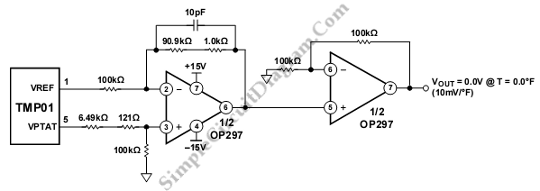# TMP01 Fahrenheit Scale Temperature Sensor

This schematic diagram is about TMP01 Fahrenheit Scale Temperature Sensor circuit. This circuit is used to convert VPTAT into an output that can be read directly in degrees Fahrenheit. The scale of this circuit is 10 mV/°F. This circuit consist of two stage,s first stage is differentiating circuit that has only unity gain or less. Then, the second stage is amplifier that gives a gain of two. The second stage is used to complete the conversion to the Fahrenheit scale. Here is the schematic diagram of the circuit:When the temperature is 0.0°F, the output voltage is 0.00V. the Output voltage is 700 mV for room temperature (70°F). The operating range of this circuit is -40°F to +185°F. The errors of this circuit is caused by the inaccuracies of the resistor values. [Circuit’s schematic diagram source: analog.com]